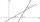# Analytic geometry + cosine - math problems

#### Number of problems found: 12

• CuboidsTwo separate cuboids with different orientation in space. Determine the angle between them, knowing the direction cosine matrix for each separate cuboid. u1=(0.62955056, 0.094432584, 0.77119944) u2=(0.14484653, 0.9208101, 0.36211633)
• Angle of the body diagonalsUsing vector dot product calculate the angle of the body diagonals of the cube.
• Angle between vectorsFind the angle between the given vectors to the nearest tenth of a degree. u = (-22, 11) and v = (16, 20)
• Angle between linesCalculate the angle between these two lines: p: -8x +4y +5 =0 q: 10x +10y -7=0
• Vector sumThe magnitude of the vector u is 12 and the magnitude of the vector v is 8. Angle between vectors is 61°. What is the magnitude of the vector u + v?
• Three vectorsThe three forces whose amplitudes are in ratio 9:10:17 act in the plane at one point to balance. Determine the angles of each two forces.
• ForcesIn point O acts three orthogonal forces: F1 = 20 N, F2 = 7 N, and F3 = 19 N. Determine the resultant of F and the angles between F and forces F1, F2, and F3.
• Coordinates of square verticesI have coordinates of square vertices A / -3; 1/and B/1; 4 /. Find coordinates of vertices C and D, C 'and D'. Thanks Peter.
• Resultant forceCalculate mathematically and graphically the resultant of three forces with a common center if: F1 = 50 kN α1 = 30° F2 = 40 kN α2 = 45° F3 = 40 kN α3 = 25°
• TrianglePlane coordinates of vertices: K[11, -10] L[10, 12] M[1, 3] give Triangle KLM. Calculate its area and its interior angles.
• BearingA plane flew 50 km on a bearing 63°20' and the flew on a bearing 153°20' for 140km. Find the distance between the starting point and the ending point.
• Two forcesThe two forces F1 = 580N and F2 = 630N, have an angle of 59 degrees. Calculate their resultant force, F.

We apologize, but in this category are not a lot of examples.
Do you have an interesting mathematical word problem that you can't solve it? Submit a math problem, and we can try to solve it.

We will send a solution to your e-mail address. Solved examples are also published here. Please enter the e-mail correctly and check whether you don't have a full mailbox.

Please do not submit problems from current active competitions such as Mathematical Olympiad, correspondence seminars etc...

Line slope calculator is helpful for basic calculations in analytic geometry. The coordinates of two points in the plane calculate slope, normal and parametric line equation(s), slope, directional angle, direction vector, the length of the segment, intersections of the coordinate axes, etc. Analytic geometry - math problems. Cosine - math problems.